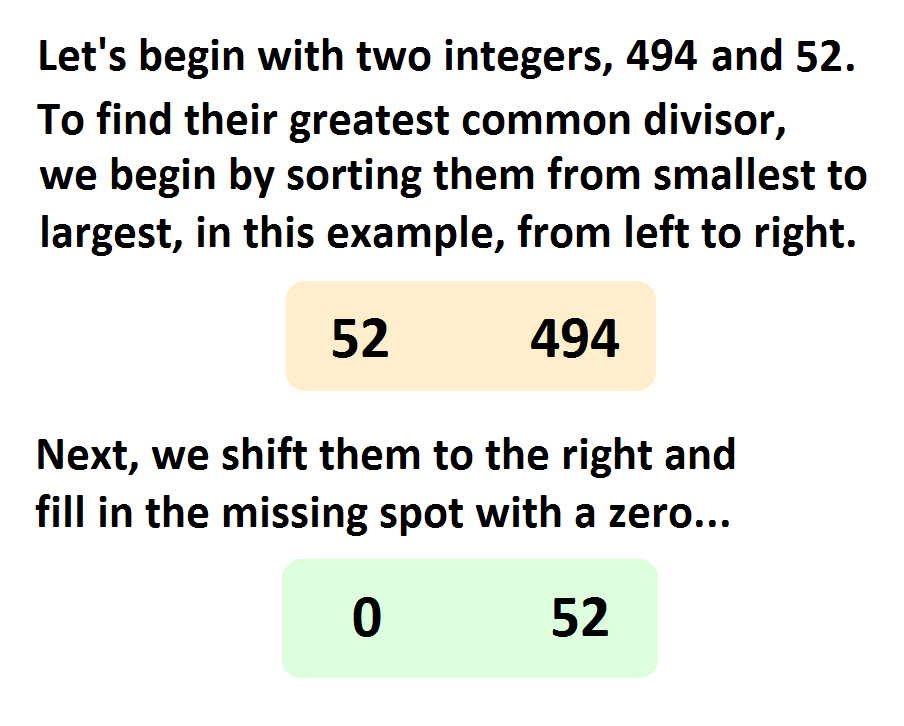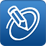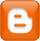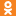# Stepwise Progression of the Expanded Euclidean Algorithm in PHPHINT: The modulo % operator yields the remainder of division and the quotient is ignored. HENCE: 5 ÷ 2 = 2.5, but 5 % 2 = 1 {1 remaining after dividing 5 by 2}.                         Refresh this page.

' . "\n"; echo '' . "\n"; echo '' . "\n"; echo '
' . "\n"; echo \$terms[\$i] . "\n"; } else { \$booboo = false; } } ?>
multiply by ' . "\n"; echo \$terms[\$i] . "\n"; } ?>
sort from left to right ' . "\n"; echo \$terms[\$i] . "\n"; } ?>
shift to the right 0 ' . "\n"; echo \$remains[\$i + 1] . "\n"; } ?>
modulo remainder 1) //for (\$q = 0; \$q < 100; \$q++) { \$cownt = \$kount; for (\$i = 0; \$i < \$save_cownt; \$i++) { if (\$i == 0) { \$remains[\$i] = \$terms[\$i]; } else { \$remains[\$i] = \$terms[\$i] % \$remains[\$i]; } // print out \$remains if (\$i % 2 == 0) { \$color_cyan = 'a0ffff'; } else { \$color_cyan = 'd5ffff'; } echo '' . "\n"; if (\$terms[\$i] != \$numeric_padding) { echo \$remains[\$i] . "\n"; } else { echo ' ' . "\n"; } } //if (\$q == 3) { break; } for (\$v = 0; \$v < \$save_cownt; \$v++) { \$terms[\$v] = \$numeric_padding; } \$kount = 0; for (\$i = 0; \$i < \$cownt; \$i++) { if (\$remains[\$i] != 0) { \$terms[\$i] = \$remains[\$i]; \$kount++; } } if (\$kount > 1) { sort (\$terms, SORT_NUMERIC); echo '
' . "\n"; echo 'sort from left to right' . "\n"; // print out \$terms for (\$v = 0; \$v < \$save_cownt; \$v++) { if (\$v % 2 == 0) { \$color_lime = 'b3ffb3'; } else { \$color_lime = 'ddffdd'; } echo '' . "\n"; if (\$terms[\$v] != \$numeric_padding) { echo \$terms[\$v] . "\n"; } else { echo ' ' . "\n"; } } echo '
' . "\n"; echo 'shift to the right' . "\n"; echo '' . "\n"; echo '0' . "\n"; \$remains = 0; // print out prior \$remains shifted to the right for (\$v = 1; \$v < \$save_cownt; \$v++) { if (\$v % 2 == 0) { \$color_lime = 'b3ffb3'; } else { \$color_lime = 'ddffdd'; } echo '' . "\n"; if (\$terms[\$v] != \$numeric_padding) { \$remains[\$v] = \$terms[\$v - 1]; echo \$remains[\$v] . "\n"; } else { echo ' ' . "\n"; } } echo '
' . "\n"; echo 'modulo remainder' . "\n"; //if (\$q == 5) { break; } // echo '*' . \$kount . '*'; } } echo '
' . "\n"; ?>

## The GCD of ' . \$holds[\$v] . ', '; } else if (\$v == (\$save_cownt - 2)) { echo '' . \$holds[\$v] . ' and '; } else if (\$v == (\$save_cownt - 1)) { echo '' . \$holds[\$v] . ''; } } ?> is .TweetThe source code in PHP for this file is here...

Up until now, the only way to deal with finding the GCD among a set of integers of greater quantity than two or three was to prime factor each of them and compare for similarities, or else compute the GCD for each and every combinatorial pair and then choose the smallest result. This expanded algorithm of Euclid provides for a more integrated approach for sets of integers greater than two.

Any version of the Euclidean Algorithm, whether it is the traditional approach of restricting ourselves to finding the GCD of only a pair of numbers, or this integrated approach among sets of numbers of indefinite quantity, hinge upon the properties which went into the construction of the Fibonacci series of numbers – but not merely the Fibonacci numbers, themselves, because the Fibonacci numbers are merely a quadratic special case of all Golden Series of Numbers stretching up to infinity of breadth. This expanded approach to finding the GCD exemplifies this breadth of complexity beyond mere pairs of numbers, because what we have overlooked up until now is that the structure of Golden Numbers Series are two-dimensional – not one-dimensional. That's why this expanded algorithm exhibits, very obviously, a tablature arrangement unlike the Fibonacci series which appears to be a mere linear progression. But to know this requires a study of the full range of Golden Ratio's existence. For that discussion, please refer to Infinite PHI.

For a really good demonstration of the traditionally restricted approach, please see this javascript method.

## Division by Zero is Not the Problem; Division is the Problem!

It could be said that defining division by zero is analogous to defining a division that results in an irrational fraction, or a repeating non-irrational fraction, because we'll always be left with a remainder no matter how accurate we try to approximate our quotient.

But better than this is to simply ignore division altogether and claim that we are performing repetitive subtractions. Now, we have to ignore neither the quotient, nor the remainder. We have to take both into consideration and give them equal merit. Whew! Doesn't peaceful coexistence feel really, really good!?

Some my want to claim that we don't get anything useful out of performing zero modulo operation upon a non-zero integer since our remainder is equal to the dividend. But we will require this when we want to get the Greatest Common Divisor from among a set of integers greater than two without resorting to any shortcuts, such as: taking them in pairs at a time, or resorting to prime factorization.

So, I think we've underestimated the significance of defining division by zero in terms of repetitive subtractions probably due to no one studying the bitwise operations of machine language computer programming, or reading Euclid's "Elements" to discover how he defined his Algorithm for finding the Greatest Common Divisor.

In other words...

```GCD(a1, a2, a3, etc)

If ax != 0

AND

ax != ay for x and y and z, etc...
```

In plain English, no integers are zero and none of them are equal to each other...

For example...

`GCD(494, 52) = 26`

Step #1. Pick a direction in which to always sort these non-zero integers from smallest to largest; I'll choose from left to right...

`52      494`

Step #2. Shift these integers over one place to the right...

` *       52`

Step #3. Fill in the missing first position with a zero...

` 0       52`

Step #4. Place step #3 underneath step #1...

```52       494
0        52
```

Step #5. Perform modulo downwards on each column...

```52       494
%0       %52
------------
52        26
```

Step #6. Repeat from step #1 until all but one column zeros out.

```26       52
%0      %26
-----------
26        0
```

Step #7. The last remaining column (containing a non-zero integer) is practically the answer, because it will repeat itself, indefinitely, if we didn't call it quits at this point.

Let's take another example...

```GCD(99, 9, 33) = 3

9      33       99
%0      %9      %33
-------------------
9       6        0

6       9
%0      %6
----------
6       3

3       6
%0      %3
----------
3       0
```

But let's do it the other way of not letting any columns disappear...

```GCD(99, 9, 33) = 3

9      33       99
%0      %9      %33
-------------------
9       6        0

0       6        9
%0      %0       %6
-------------------
0       6        3

0       3        6
%0      %0       %3
-------------------
0       3        0
```

Either way works...

So, I've just demonstrated the philosophical practicality of performing repetitive subtractions, and thus avoid the quandry of dividing by zero, by showing a concrete example of performing a non-linear variety of the Euclidean Algorithm on any set of integers greater than two in such a way that requires that we perform what appears to be division by zero while ignoring the quotient. Ignoring quotients doesn't mean that the division never occurred or else I'd never get to propel this expanded variety of the Euclidean Algorithm to its ultimate fruition!

Here is the above exampled as a JavaScript file,

gcd.js

sTaTs